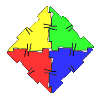#### You may also like### Geoboards

This practical challenge invites you to investigate the different squares you can make on a square geoboard or pegboard.### Polydron

This activity investigates how you might make squares and pentominoes from Polydron.If you had 36 cubes, what different cuboids could you make?

# This Pied Piper of Hamelin

##### Age 7 to 11Challenge Level

We had a good number of solutions sent in, thank you.  Here we will feature those of you who've looked at many possibilities. From George at Linton Heights Junior School”¨ we had the following:

a) $0$ rats and $300$ children
b) $1$ rat and $298$ children
c) $5$ rats and $290$ children
d) $10$ rats and $280$ children
e) $25$ rats and $250$ children
f) $50$ rats and $200$ children
g) $100$ rats and $100$ children
h) $125$ rats and $50$ children
i) $150$ rats and $0$ children

From Patrick at Manorcroft Primary School we had this good explanation, well done:”¨

There are $148$ different combinations of child and rat because I figured out that you could replace $1$ rat with $2$ children (because one rat has twice as many legs as a child) so the maximum possible children to rats are $298$ children to $1$ rat and the maximum possible rats to children is $149$ rats to $2$ children. If you take $1$ from $149$ to get $148$ possiblities.

Year $5”¨$ pupils from St Ambrose Catholic Primary School said;
”¨

There are many possible answers to this question, to find out how many children, you can start with the number of rats. You can go from $1$ rat to $149$ rats to work out how many children.  The rule is: multiply by $4$, take away from $600$, divide by $2$.
For example, $1$ rat= $298$ children because $1$x$4=4 600-4=596, 596$ divided by $2=298$.
If we start with the number of children the rule is: multiply by $2$, take away from $600$, divide by $4$.
For example, $2$ children $= 149$ rats because $2$x$2=4$, $600-4= 596, 596$ divided by $4= 149$.
There are some patterns that we noticed, such as: If you take $1$ away from the amount of rats, it adds $2$ to the amount of children. For example: $86$ children, $107$ rats; $106$ rats, $88$ children.

Matthew from Calcot Junior School also discovered this general rule. He said:

There is a formula which is however many rats you have, double that number and take it off 300. Your result is how many children there are.

The total number of legs is 600. Each child has 2 legs, each rat has 4. So the total number of legs can be written as:

Legs = Children x 2 + Rats x 4

That's very helpful, Matthew. By 'children', I think Matthew means the number of children, and 'rats' means the number of rats.

Because we know the number of legs, we can write:

600 = Children x 2 + Rats x 4

Then if we know either children or rats, we can use this to work out the missing one. For example, if there are 100 Children:

600 = 100 x 2 + Rats x 4
600 = 200 + Rats x 4
400 = Rats x 4

Rats = 100

So Rats = (Legs - 2 x Children) $\div$ 4

If you know legs and rats, to work out children:

Children = (Legs - 4xRats) $\div$ 2

This is extremely well explained, thank you Matthew. Matthew calculated all the possible answers, which you can see here. Thank you for sharing this with us, Matthew.

James”¨, who calls his school  "BG"”¨ sent in the following good explanation:

When working out the pattern for children/rats, I thought it would be a good idea to start with either everything as children or everything as rats. I decided to start with everything as children, which would be $300$ children and $0$ rats, since $600$ halved is $300$.

Then, I knew if I wanted to get all possible solutions, I'd need to come up with a pattern. My pattern was adding some of the children's legs, to the rats each time. But of course, since rats have twice as many legs as children, that wouldn't work, so I took away two children for every rat I added, as shown in the pattern below:

 Children Rats Legs $300$ $0$ $600 + 0$ $298$ $1$ $596+ 4$ $296$ $2$ $592 + 8$ $294$ $3$ $588 + 12$

I did that on and on, until I was certain, that every time, the legs would total up to $600$. By looking at the number of children, and noticing that it started at $300$, and got $2$ fewer each time, I divided $300$ by $2$, and then added on the $1$ possibilty, with $0$ children, to work out there would be $151$ possible solutions for this.

These solutions are really good. Well done, keep submitting solutions to other activities.